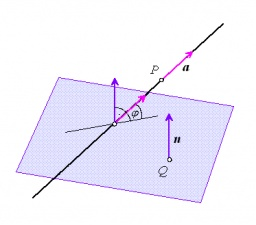# Parametrically 6400

Find the angle of the line, which is determined parametrically x = 5 + t y = 1 + 3t z = -2t t belongs to R and the plane, which is determined by the general equation 2x-y + 3z-4 = 0.

X =  30 °

### Step-by-step explanation:Did you find an error or inaccuracy? Feel free to write us. Thank you!

Tips for related online calculators
Line slope calculator is helpful for basic calculations in analytic geometry. The coordinates of two points in the plane calculate slope, normal and parametric line equation(s), slope, directional angle, direction vector, the length of the segment, intersections of the coordinate axes, etc.
Our vector sum calculator can add two vectors given by their magnitudes and by included angle.
The Pythagorean theorem is the base for the right triangle calculator.